• 集算器
教程
函数参考
用户参考
外部库使用指南
数据文件工具用户参考
DQL教程
集群管理器使用说明
SPL命令执行器用户参考
• 润乾报表
教程
填报教程
分析教程
报表中心教程
用户参考
程序员参考
高级指南
通用查询
DQL部署集成手册
• 易明建模
用户参考
json参数说明
• 润乾官网

# memory()

## cs.memory(K,…)

cs.memory(K,…)

 K 键 cs 游标

 @p 用第一字段作分段，要求cs对第一个字段有序 @v 使用该选项时，cs中数据内容必须为纯序表，结果返回列式内表 @x 生成内表后自动关闭游标

 A 1 =demo.cursor("select EID,NAME,GENDER,SALARY from EMPLOYEE where EID<10") 返回取数游标 2 =A1.memory(EID) 返回键为EID的内表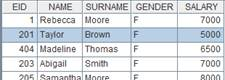A 1 =connect("demo").query("select  EID,NAME,GENDER,SALARY  from  employee").sort(EID ) 返回对EID有序的序表 2 =A1.cursor() 返回游标 3 =A2.memory@p(EID) 用A2游标生成内表，根据第一个字段EID分段

 A 1 =to(1000).new(~:ID,~*~:pNum) 生成序表，结构如下：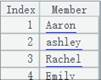2 =A1.cursor() 生成游标，游标中数据内容为纯序表 3 =A2.memory@v() 使用@v选项，生成列式内表

## cs.memory(K,…)

cs.memory(K,…)

 K 键，可省略 cs 集群游标

 A 1 =file("test0.ctx","169.254.121.62:8281") 打开集群文件 2 =A1.open() 打开集群组表 3 =A2.attach(table) 取出集群附表table 4 =A3.cursor(NAME,GENDER;EID<6) 筛选集群附表table中EID<6的NAME,GENDER列后返回成游标 5 =A4.memory(GENDER) 集群游标生成键为GENDER的集群内表

## memory(h,V)

memory(h,V)

 h 分机序列 V 内表全局变量

 A 1 =memory(["192.168.31.72:8281","192.168.31.72:8291"],arg1) 本地内表arg1拼出集群内表 2 =A1.cursor().fetch()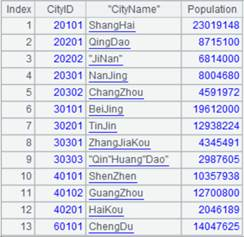## T.memory()

T.memory()

 T 序表/纯序表，如果有键和索引，将被继承

 @o 直接使用原序表的记录，原序表数据变动时，返回的内表数据跟随变动；缺省为将原序表的数据复制并转换，原数据变动时，内表数据不变

 A 1 =demo.query("select EID,NAME,GENDER,SALARY from employee where EID <= 30") 2 =A1.keys(EID) 设置A1的键为EID 3 =A1.memory() 将序表A1转换为内表，键被继承 4 =A1.memory@o() 5 =A1.insert(0) 在原序表末尾追加一条空记录。 查看A2末尾不显示空记录，A3末尾显示空记录。 6 =demo.query@v("select * from DEPT") 返回纯序表 7 =A6.memory() 将A6纯序表转换成内表，并且保持表的纯性

## T.memory(C,…;w)

T.memory(C,…;w)

 C 列名，可省略，缺省读入全部列 w 过滤条件，缺省不过滤 T 组表的实表

 @p 用第一字段作分段，要求T对第一个字段有序；当T有分段且参数C中包含T的第一个字段时，内表将继承组表T的分段属性 @v 使用该选项时，表T必须为纯序表，结果返回列式内表 @x 生成内表 自动关闭T @w 用于复组表。 按更新机制归并，分表间存在相同键值时，忽略分表号较小的分表中的记录。分段要以第1分表为准，后面有些分表可能非常小可先全读。

 A 1 =file("D:\\ employees.ctx") employees为已存在的组表文件 2 =A1.open() 打开组表 3 =A2.attach(t1) 返回组表上已存在的附表t1 4 =A2.memory() 用基表全部列生成内表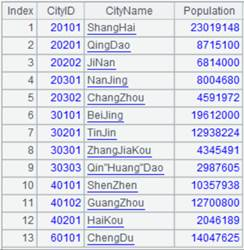5 =A2.memory(EID,Dept,Name;EID<5) 取基表的部分列并筛选出EID<5的数据生成内表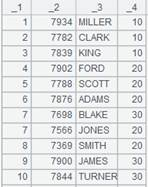6 =A3.memory() 取附表全部列生成内表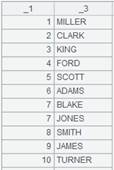A 1 =connect("demo").cursor("select  EID,NAME,GENDER,SALARY  from  employee").sortx(EID ) 返回游标，游标中数据对EID有序 2 =file("emp.ctx") 生成组表文件 3 =A2.create@yp(#EID,NAME,GENDER,SALARY) 创建组表的基表，并且将第一个字段作为分段键 4 =A3.append@i(A1) 将游标中的数据追加到基表中 5 =A2.open() 打开组表文件 6 =A5.memory@p(EID,NAME) 用实表A5生成内表，使用@p选项，将第一个字段作为分段键，组表T有分段且A6的读取的字段中包含第一个字段EID，所以内表会继承组表A5的分段属性

 A 1 =to(1000).new(~:ID,~*~:pNum).cursor() 返回游标,游标中的数据为纯序表，表结构如下：2 =file("tb1.ctx") 生成组表文件 3 =A2.create@y(#ID,pNum) 创建组表的基表 4 =A3.append@i(A1) 将游标中的数据追加到基表中 5 =A4.memory@v() 使用@v选项，结果返回列式内表

 A 1 =connect("demo").cursor("select  EID,NAME,GENDER  from employee") 返回游标，游标内容如下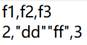2 =file("em.ctx":[1,2]) 文件组1.em.ctx、2.em.ctx 3 =A2.create@y(#EID,NAME,GENDER;if(GENDER=="F",1,2)) 创建复组表，EID为键，GENDER值为F的记录分到1.em.ctx中，其余分到2.em.ctx中 4 =A3.append@ix(A1) 将A1游标中的数据追加到复组表中 5 =create(EID,NAME,GENDER).record([1,"AAA","M"]).cursor() 返回游标，内容如下：6 =file("em.ctx":) 7 =A6.create@y(#EID,NAME,GENDER;3) 增加分表3.em.ctx 8 =A7.append@i(A5) 将A5游标中的内容追加到分表3.em.ctx中，此时分表1.em.ctx、3.ec.ctx中都存在主键为的记录 9 =file("em.ctx":[1,2,3]).open() 打开复组表em.ctx 10 =A9.memory() 用复组表生成内表：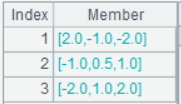11 =A9.memory@w() 用复组表生成内表，使用@w选项，按照更新机制归并，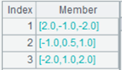## T.memory(C,…;w)

T.memory(C,…;w)

 C 列名，可省略，省略读入全部列 w 过滤条件，缺省不过滤 T 有维的集群组表的实表

 A 1 =file("emp1.ctx",["192.168.0.116:8281","192.168.0.129:8281"]) 打开集群文件 2 =A1.open() 产生集群组表 3 =A2.attach(t1) 取出集群附表t1 4 =A3.memory(NAME,GENDER;EID<5) 通过表t1生成列为NAME，GENDER的集群内表，筛选条件为EID<5